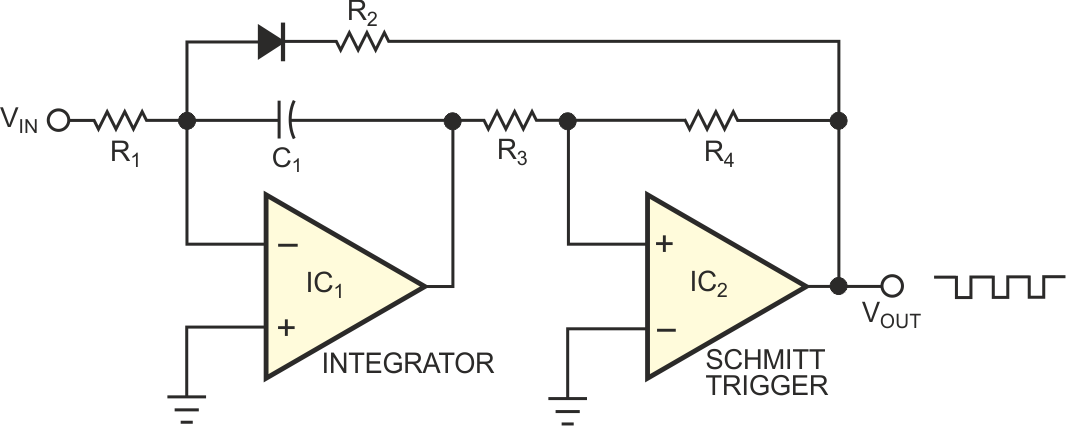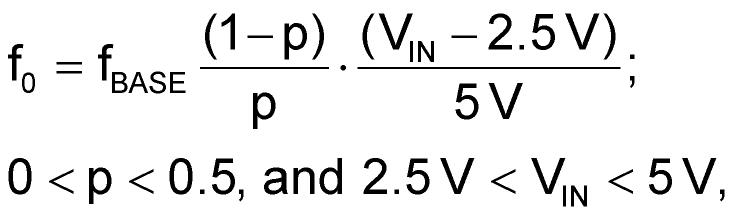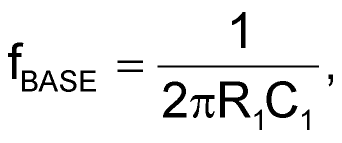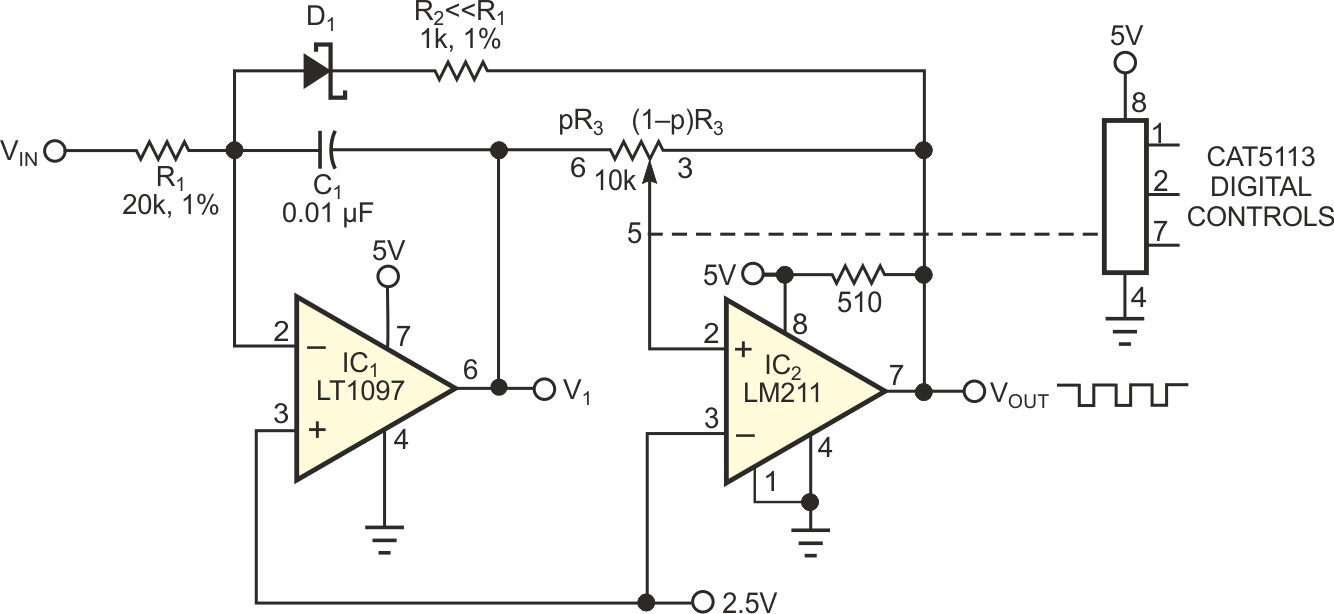# DPP adds versatility to VFC

## ON Semiconductor CAT5113

Chuck Wojslaw

EDNFigure 1. This schematic depicts a basic voltage-to-frequency converter.

The basic VFC (voltage-to-frequency converter) in Figure 1 comprises an integrator (IC1) and a Schmitt-trigger circuit (IC2). The integrator converts the dc input voltage, VIN, to a linear voltage ramp, and the Schmitt trigger sets the limits of the integrator's output voltage. Feedback around both circuits provides the condition for oscillation. The DPP (digitally programmable potentiometer) in Figure 2 adds programmable limits to the Schmitt trigger and adds two powerful features to the VFC. First, the scale or conversion factor is programmable, and, second, for a fixed dc-input voltage, the converter is a programmable oscillator. The frequency, f0, of the single-supply converter in Figure 2 is:whereand p is the relative position of the wiper from one end (0) of the DPP to the other end (1). For the100-tap CAT5113 potentiometer, the range of the scale-factor term (1–p)/p is 1 to 99 with a resolution and accuracy of approximately 1%. For the values shown in Figure 2, the practical range of frequencies is 500 Hz to 25 kHz. Higher bandwidth, rail-to-rail CMOS versions of IC1 and IC2, and a greater R1/R2 ratio can extend the accuracy and range of the circuit. The automated, accurate setting of the scale factor saves manufacturing test time and eliminates the need for expensive, accurate resistors and capacitors. The scale factor relates to the ratiometric temperature coefficient of the DPP and hence is minimally temperature-dependent. You can use the circuit as a programmable oscillator when VIN is fixed and the potentiometer's wiper setting changes the limits of the Schmitt trigger.Figure 2. Using a digitally programmable potentiometer, you can vary the scale factor of this voltage- to-frequency converter.

EDN